# Triangle

Determine if it is possible to construct a triangle with sides 28 31 34 by calculation.

Result

#### Solution:Leave us a comment of example and its solution (i.e. if it is still somewhat unclear...):Be the first to comment!## Next similar examples:

1. TriangleProve whether you can construct a triangle ABC, if a=9 cm, b=10 cm, c=4 cm.
2. Triangle P2Can triangle have two right angles?
3. Circles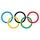For the circle c1(S1; r1=146 cm) and c2(S2; r2 = 144 cm) is distance of centers |S1S2| = 295 cm. Determine the distance between the circles.
4. CagesHonza had three cages (black, silver, gold) and three animals (guinea pig, rat and puppy). There was one animal in each cage. The golden cage stood to the left of the black cage. The silver cage stood on the right of the guinea pig cage. The rat was in the
5. Compare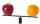Compare with characters >, <, =: 85.57 ? 80.83
6. Foot in busIt was 102 people on the bus. 28 girls had two dogs. A 11 girls had one dog. At the next stop seceded 5 dogs (even with their owners). They got two boys together with three dogs. The bus drove one driver. How many foot were in bus?Added together and write as decimal number: LXVII + MLXIV
8. Expressions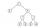Find out value of expressions if a = -1, b =2: x=b - 2a - ab y=a3 - b2 - 2ab z=a2 b3 - a3 b2 w=a + b + a3 - b2
9. Valid numberRound the 453874528 on 2 significant numbers.
10. Eq1Solve equation: 4(a-3)=3(2a-5)
11. Ten pupils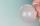10 pupils went to the store. 6 pupils bought lollipops and 9 pupils bought chewing gum. How many pupils have bought both lollipops and chewing gums (if everyone bought something)?
12. Plane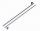On how many parts divide plane 6 parallels?
13. Six te 2If 3t-7=5t, then 6t=
14. Angles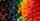Which of those angles are obtuse?
15. If-then equationIf 5x - 17 = -x + 7, then x =
16. Line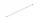How many parts of line divide 5 (different) points that lie on it?
17. Math classificationIn 3A class are 27 students. One-third got a B in math and the rest got A. How many students received a B in math?